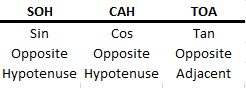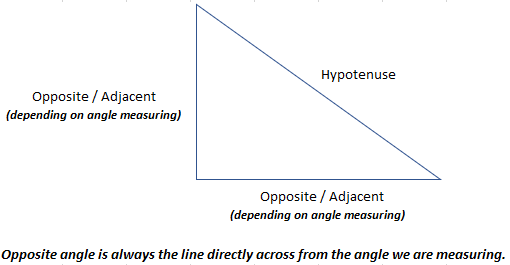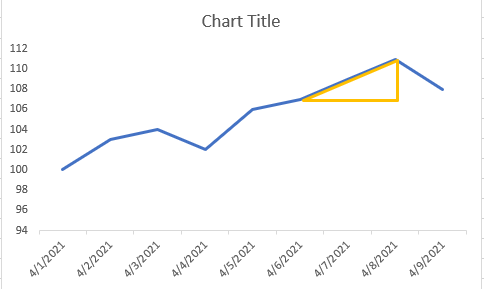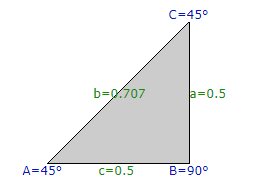# How To Find Slope of Line In ThinkOrSwim

#### Internet Name

##### Member
I wanted to play with the angle of a moving average line as an indicator since there seems to be a pretty distinct pattern with them. Unfortunately, the answer of "how to calculate the slope of a moving average line" was not as readily available as I would've wished. It took me a couple days to figure out how to code thinkscript to get it to return the angle. I'd like to share the process and the final code. If anyone ends up incorporating this into their thinkscript codes, I'd love to hear about it if you wish to share.

We have the use the trigonometric functions to determine what the slope is. I ended up having to go back and learn basic trigonometry that I know I ignored in High School. When we go to the ThinkScript guide, there are COS, SIN, and TAN functions, but the guide is very brief on what they do or what the number being returned means.In a stock chart, the only side lengths we know are the opposite and adjacent. The hypotenuse is what we are solving for. So the TAN function is the only function we can use.We're going to measure the lower left angle, so the stock price is the opposite side, and the date is the adjacent side. With the adjacent side, we need to make sure that all stocks are on an even playing field by using percentages. For the periods on the adjacent side, I've used a value of 0.5 per period so that a percent increase of 50% will show a 45 degree angle.A difficult part of the process was figuring out the difference between tangent vs. arc tangent:
• Tangent = use when you have the degree already and want to find acceptable side lengths.
• Arc Tangent = use when you have the side lengths and want to find the degree of the angle.

So we use the function "ATAN" rather than TAN. If you go into the documentation for this function and look at the example, you'll see a "180 / Double.Pi" inside ATAN and "DoublePi * 180" inside the TAN function. This is the piece we need to bridge between the lengths and the angle.

Once we do the opposite side length / adjacent side length and get the answer, we can then do the following to get the angle:
• On the calculator: find the trigonometry functions, then find and select Tan^-1. This will return the angle.
• In thinkscript: ATAN(opposite/adjacent) * 180 / Double.Pi

Final code:
Code:
``````Declare lower;
input HowManyPeriodsBackForSlopeMeasure= 1;
input SMALength = 9;
input agg = aggregationPeriod.Day;
input ShowTroubleshootLabels= no;
def SMA = Average(close(period=agg),SMALength);
def opposite = (SMA - SMA[HowManyPeriodsBackForSlopeMeasure])/SMA[HowManyPeriodsBackForSlopeMeasure];
def adjacent = HowManyPeriodsBackForSlopeMeasure * .5;
def tan = opposite / adjacent;

plot angle = ROUND(atan(tan)* 180 / Double.Pi,2);

# ---- Troubleshooting Labels ----
AddChartBubble(SecondsTillTime(1200)==0,if ShowTroubleshootLabels then angle else Double.NaN,angle,color.white);

# ---- Calculator Instructions ----
#With tan entered into the calculator, hit trigonometry, 2nd, and then tan^-1 to get the angle.``````

After testing this, I believe this is pretty accurate. When you use it on moving averages, the slopes can look alot steeper than what the degree is returning. But I believe that has to do with perspective. You can zoom in and out and the slope of the line changes, sometimes the charts start at numbers other than zero, so they can look extreme. But using the methods discussed above puts the stocks on an even playing field I believe. If you do want to turn up the sensitivity, reducing the 180 in "180 / Double.Pi" would seem to be the easiest way.

•••quantumomegallc, Teknical, trendr and 5 others
Changing the bottom length (adjacent side) of the triangle from .5 to something like .1 gives a little more distinction between the angle numbers.

I posted this on another forum not so long ago... I'll think through your post a bit more over a coffee later this morning.

Angles are funny things. To say that an angle is 45° is also to say that for every unit to the right, the line moves 1 unit up. Since our x axis units are time (often minutes) and our y axis are price (usually dollars), would a 45° angle be \$1 per minute? 1¢ per 5 minutes...? You see where this is going. Expressing slopes in dollars per hour might be useful, but then so might ticks per 500 trades. As long as you are comparing apples to apples you should be ok. Figure out what ToS is giving you for units and what you're calculating and then decide what you want to see.

EDIT:
This is taken directly from the docs here:
https://tlc.thinkorswim.com/center/reference/thinkScript/Functions/Math---Trig/ATan
and will give you the slope in degrees of a line with a known x and y (run and rise, and in our case length and change in price). Such that over three bars (that's the length and the x axis value) the change (here our y axis and change in price) is the SMA[final] - SMA[initial]. The ATAN function returns the value in radians, which are converted to degrees.

Code:
``````declare lower;
input length = 3;
def avg = Average(close, length);
def height = avg - avg[length];
plot "Angle, deg" = ATan(height/length) * 180 / Double.Pi;``````

Mashume

Last edited:
••Gordon, netarchitech, halcyonguy and 1 other person
I've been comparing it to just using the change % of the moving average line, and they both seem to be identical. So maybe it was all for naught. I'll have to play around with them and see if there are any advantages or disadvantages.

Code:
``````declare lower;
input length = 9;
input agg = aggregationPeriod.Day;

def avg1 = Average(close(period=agg), length);
#plot avg = avg1-avg1;
plot zerobase = 0;
Zerobase.SetDefaultColor(Color.white);
Zerobase.SetStyle(Curve.Short_Dash);
plot NumChange;
NumChange = (avg1-avg1)/avg1 *100;``````

••dudemanguy, netarchitech, Mr_Wheeler and 3 others
I'm currently trying to figure out how ThinkOrSwim calculates the greeks and Implied Volatility. If anyone has any insight on how it does this and would share, I would be very greatful.

here are some links on option greeks that might help

options data, greeks, delta, theta,

custom
Black Scholes Options Model by Mobius for ThinkorSwim
markos
Jul 10, 2019

post7
Here are some threads that mention the SeriesVolatility function
https://www.one-tab.com/page/jQhBYwoGTi-zEaCB7pMgjQ

--------------------------------

indicators
Option Greeks Calculation Labels for ThinkorSwim
markos
Jul 25, 2019

===========================
options, 2nd order greeks

vanna
https://spotgamma.com/options-vanna-charm/

https://www.thebalance.com/vanna-explanation-of-the-options-greek-1031331

2nd order greeks

Options Greeks: Vanna, Charm, Vomma, DvegaDtime

https://spotgamma.com/options-vanna/

https://financetrainingcourse.com/education/2014/06/vega-volga-and-vanna-the-volatility-greeks/

option basics
https://www.thebalance.com/options-definition-3305952

------------------------
xls file
calc greeks
https://financetrainingcourse.com/e...ks-using-solver-to-hedge-vega-gamma-exposure/
In this chapter we start with building a simple Excel spreadsheet that will us allow us to hedge Gamma and Vega exposure for a single short position in a call option contract.

•mashume
I wanted to play with the angle of a moving average line as an indicator since there seems to be a pretty distinct pattern with them. Unfortunately, the answer of "how to calculate the slope of a moving average line" was not as readily available as I would've wished. It took me a couple days to figure out how to code thinkscript to get it to return the angle. I'd like to share the process and the final code. If anyone ends up incorporating this into their thinkscript codes, I'd love to hear about it if you wish to share.

We have the use the trigonometric functions to determine what the slope is. I ended up having to go back and learn basic trigonometry that I know I ignored in High School. When we go to the ThinkScript guide, there are COS, SIN, and TAN functions, but the guide is very brief on what they do or what the number being returned means.In a stock chart, the only side lengths we know are the opposite and adjacent. The hypotenuse is what we are solving for. So the TAN function is the only function we can use.We're going to measure the lower left angle, so the stock price is the opposite side, and the date is the adjacent side. With the adjacent side, we need to make sure that all stocks are on an even playing field by using percentages. For the periods on the adjacent side, I've used a value of 0.5 per period so that a percent increase of 50% will show a 45 degree angle.A difficult part of the process was figuring out the difference between tangent vs. arc tangent:
• Tangent = use when you have the degree already and want to find acceptable side lengths.
• Arc Tangent = use when you have the side lengths and want to find the degree of the angle.

So we use the function "ATAN" rather than TAN. If you go into the documentation for this function and look at the example, you'll see a "180 / Double.Pi" inside ATAN and "DoublePi * 180" inside the TAN function. This is the piece we need to bridge between the lengths and the angle.

Once we do the opposite side length / adjacent side length and get the answer, we can then do the following to get the angle:
• On the calculator: find the trigonometry functions, then find and select Tan^-1. This will return the angle.
• In thinkscript: ATAN(opposite/adjacent) * 180 / Double.Pi

Final code:
Code:
``````Declare lower;
input HowManyPeriodsBackForSlopeMeasure= 1;
input SMALength = 9;
input agg = aggregationPeriod.Day;
input ShowTroubleshootLabels= no;
def SMA = Average(close(period=agg),SMALength);
def opposite = (SMA - SMA[HowManyPeriodsBackForSlopeMeasure])/SMA[HowManyPeriodsBackForSlopeMeasure];
def adjacent = HowManyPeriodsBackForSlopeMeasure * .5;
def tan = opposite / adjacent;

plot angle = ROUND(atan(tan)* 180 / Double.Pi,2);

# ---- Troubleshooting Labels ----
AddChartBubble(SecondsTillTime(1200)==0,if ShowTroubleshootLabels then angle else Double.NaN,angle,color.white);

# ---- Calculator Instructions ----
#With tan entered into the calculator, hit trigonometry, 2nd, and then tan^-1 to get the angle.``````

After testing this, I believe this is pretty accurate. When you use it on moving averages, the slopes can look alot steeper than what the degree is returning. But I believe that has to do with perspective. You can zoom in and out and the slope of the line changes, sometimes the charts start at numbers other than zero, so they can look extreme. But using the methods discussed above puts the stocks on an even playing field I believe. If you do want to turn up the sensitivity, reducing the 180 in "180 / Double.Pi" would seem to be the easiest way.

Let me know if you find this useful or anything else to note.

I'm currently trying to figure out how ThinkOrSwim calculates the greeks and Implied Volatility. If anyone has any insight on how it does this and would share, I would be very greatful.

I've been comparing it to just using the change % of the moving average line, and they both seem to be identical. So maybe it was all for naught. I'll have to play around with them and see if there are any advantages or disadvantages.

Code:
``````declare lower;
input length = 9;
input agg = aggregationPeriod.Day;

def avg1 = Average(close(period=agg), length);
#plot avg = avg1-avg1;
plot zerobase = 0;
Zerobase.SetDefaultColor(Color.white);
Zerobase.SetStyle(Curve.Short_Dash);
plot NumChange;
NumChange = (avg1-avg1)/avg1 *100;``````
I wanted to play with the angle of a moving average line as an indicator since there seems to be a pretty distinct pattern with them. Unfortunately, the answer of "how to calculate the slope of a moving average line" was not as readily available as I would've wished. It took me a couple days to figure out how to code thinkscript to get it to return the angle. I'd like to share the process and the final code. If anyone ends up incorporating this into their thinkscript codes, I'd love to hear about it if you wish to share.

We have the use the trigonometric functions to determine what the slope is. I ended up having to go back and learn basic trigonometry that I know I ignored in High School. When we go to the ThinkScript guide, there are COS, SIN, and TAN functions, but the guide is very brief on what they do or what the number being returned means.In a stock chart, the only side lengths we know are the opposite and adjacent. The hypotenuse is what we are solving for. So the TAN function is the only function we can use.We're going to measure the lower left angle, so the stock price is the opposite side, and the date is the adjacent side. With the adjacent side, we need to make sure that all stocks are on an even playing field by using percentages. For the periods on the adjacent side, I've used a value of 0.5 per period so that a percent increase of 50% will show a 45 degree angle.A difficult part of the process was figuring out the difference between tangent vs. arc tangent:
• Tangent = use when you have the degree already and want to find acceptable side lengths.
• Arc Tangent = use when you have the side lengths and want to find the degree of the angle.

So we use the function "ATAN" rather than TAN. If you go into the documentation for this function and look at the example, you'll see a "180 / Double.Pi" inside ATAN and "DoublePi * 180" inside the TAN function. This is the piece we need to bridge between the lengths and the angle.

Once we do the opposite side length / adjacent side length and get the answer, we can then do the following to get the angle:
• On the calculator: find the trigonometry functions, then find and select Tan^-1. This will return the angle.
• In thinkscript: ATAN(opposite/adjacent) * 180 / Double.Pi

Final code:
Code:
``````Declare lower;
input HowManyPeriodsBackForSlopeMeasure= 1;
input SMALength = 9;
input agg = aggregationPeriod.Day;
input ShowTroubleshootLabels= no;
def SMA = Average(close(period=agg),SMALength);
def opposite = (SMA - SMA[HowManyPeriodsBackForSlopeMeasure])/SMA[HowManyPeriodsBackForSlopeMeasure];
def adjacent = HowManyPeriodsBackForSlopeMeasure * .5;
def tan = opposite / adjacent;

plot angle = ROUND(atan(tan)* 180 / Double.Pi,2);

# ---- Troubleshooting Labels ----
AddChartBubble(SecondsTillTime(1200)==0,if ShowTroubleshootLabels then angle else Double.NaN,angle,color.white);

# ---- Calculator Instructions ----
#With tan entered into the calculator, hit trigonometry, 2nd, and then tan^-1 to get the angle.``````

After testing this, I believe this is pretty accurate. When you use it on moving averages, the slopes can look alot steeper than what the degree is returning. But I believe that has to do with perspective. You can zoom in and out and the slope of the line changes, sometimes the charts start at numbers other than zero, so they can look extreme. But using the methods discussed above puts the stocks on an even playing field I believe. If you do want to turn up the sensitivity, reducing the 180 in "180 / Double.Pi" would seem to be the easiest way.

Let me know if you find this useful or anything else to note.

I'm currently trying to figure out how ThinkOrSwim calculates the greeks and Implied Volatility. If anyone has any insight on how it does this and would share, I would be very greatful.
Thank you for this coding. I am finding it useful. As the SMA crosses above the zero line (entry), the exits work well with the TMO indicator with a crossing below the zero line as the final exit. By the way, I added the following to your code to assist with the visual:

plot centerLine = 0;

Last edited by a moderator:
•Internet Name and MerryDay
This is from my post, not to take away from anything any of the other authors put in that thread, but I know what to change in what I wrote...
Code:
``````declare lower;
input length = 3;
def avg = Average(close, length);
def height = avg - avg[length];
plot "Angle, deg" = ATan(height/length) * 180 / Double.Pi;``````
in that code, there is an average of the clsoe price -- this is what we are calculating the angle of.
ToS has a function: LinearRegCurve() which will, I think, create a series of values.
We can substitute the moving average with the linear regression function:
Code:
``````declare lower;
input length = 3;
def data = LinearRegCurve(price = close, length = length);
def height = data - data[length];
plot "Angle, deg" = ATan(height/length) * 180 / Double.Pi;``````
and it should give you the slope of the regression curve in degrees.

hope that helps make some more sense of it for you.

-mashume

This is from my post, not to take away from anything any of the other authors put in that thread, but I know what to change in what I wrote...
Code:
``````declare lower;
input length = 3;
def avg = Average(close, length);
def height = avg - avg[length];
plot "Angle, deg" = ATan(height/length) * 180 / Double.Pi;``````
in that code, there is an average of the clsoe price -- this is what we are calculating the angle of.
ToS has a function: LinearRegCurve() which will, I think, create a series of values.
We can substitute the moving average with the linear regression function:
Code:
``````declare lower;
input length = 3;
def data = LinearRegCurve(price = close, length = length);
def height = data - data[length];
plot "Angle, deg" = ATan(height/length) * 180 / Double.Pi;``````
and it should give you the slope of the regression curve in degrees.

hope that helps make some more sense of it for you.

-mashume
Linear Regression Curve has a length and two angles. Where can I adjust these parameters?

Code:
``````declare lower;
input length = 9;
def agg = getaggregationPeriod();

def avg1 = LinearRegCurve(price = close, length = length);
plot zerobase = 0;
Zerobase.SetDefaultColor(Color.white);
Zerobase.SetStyle(Curve.Short_Dash);
plot NumChange;
NumChange = (avg1-avg1)/avg1 *100;``````

Change Avg1 to your moving average or curve. Then update the inputs. This will return the change % from the prior bar to the current bar. In my testing, the line is identical to the code in the first reply that he linked.

If, instead of looking at the change from prior to current, you want to look at 10 bars back to current, you can change the  in the NumChange to 10.

Edit: So the code might look like this:

Code:
``````declare lower;
input lengthOfLine = 9;           # this is the # of periods the line is using. 9SMA = 9.
def agg = getaggregationPeriod();
def HowManyBarsBack = 10;    # How many bars back is the first point to the last point. 1 is recommended if you want to view the line slope.

def avg1 = LinearRegCurve(price = close, length = length);  #This is where you change the line type.
plot zerobase = 0;
Zerobase.SetDefaultColor(Color.white);
Zerobase.SetStyle(Curve.Short_Dash);
plot NumChange;
NumChange = (avg1-avg1[HowManyBarsBack])/avg1[HowManyBarsBack] *100;``````

•High_Velocity
I don't have it open right now, but in the ThinkScript editor, you can be sure to toggle on the Reference library/Inspector part of the window and edit the parameters of the function that way. I'll post up a screen shot tomorrow. The docs on LinearRegCurve:
https://tlc.thinkorswim.com/center/reference/Tech-Indicators/studies-library/G-L/LinearRegCurve
only show two input parameters. If you're using something else, you will need to adjust your parameters.

The same code can be used to give the slope of any function... at least any regular function that is continuous and does not explode.

bro couldn't you literally just do

(y1 - y0)/(x1 - x0)

and then use a threshold in relation to that? for instance, if the slope is 1 then the angle is 45 degrees.

Very cool concept for setting profit taking areas.

bro couldn't you literally just do

(y1 - y0)/(x1 - x0)

and then use a threshold in relation to that? for instance, if the slope is 1 then the angle is 45 degrees.
That won't give you an accurate indication of degree of change. When you are comparing price (which is one scale) and time periods (1,2,3, etc.) they don't have a direct correlation. If we could assign a scale on a graph of 1:x then that would be possible, but when you are switching chart periods (5m, D, etc.) and symbols, it would be nigh unto impossible to hard code that scale into anything that would be useful. This is what @mashume was talking about in his post above. If someone who didn't struggle through calculus has a better answer, I'd welcome it, but that would be pretty advanced mathematics, imo.

### Not the exact question you're looking for?

87k+ Posts
227 Online## The Market Trading Game Changer

Join 2,500+ subscribers inside the useThinkScript VIP Membership Club
• Exclusive indicators
• Proven strategies & setups
• Private Discord community
• Exclusive members-only content
• 1 full year of unlimited support

What is useThinkScript?

useThinkScript is the #1 community of stock market investors using indicators and other tools to power their trading strategies. Traders of all skill levels use our forums to learn about scripting and indicators, help each other, and discover new ways to gain an edge in the markets.

How do I get started?

We get it. Our forum can be intimidating, if not overwhelming. With thousands of topics, tens of thousands of posts, our community has created an incredibly deep knowledge base for stock traders. No one can ever exhaust every resource provided on our site.

If you are new, or just looking for guidance, here are some helpful links to get you started.

What are the benefits of VIP Membership?